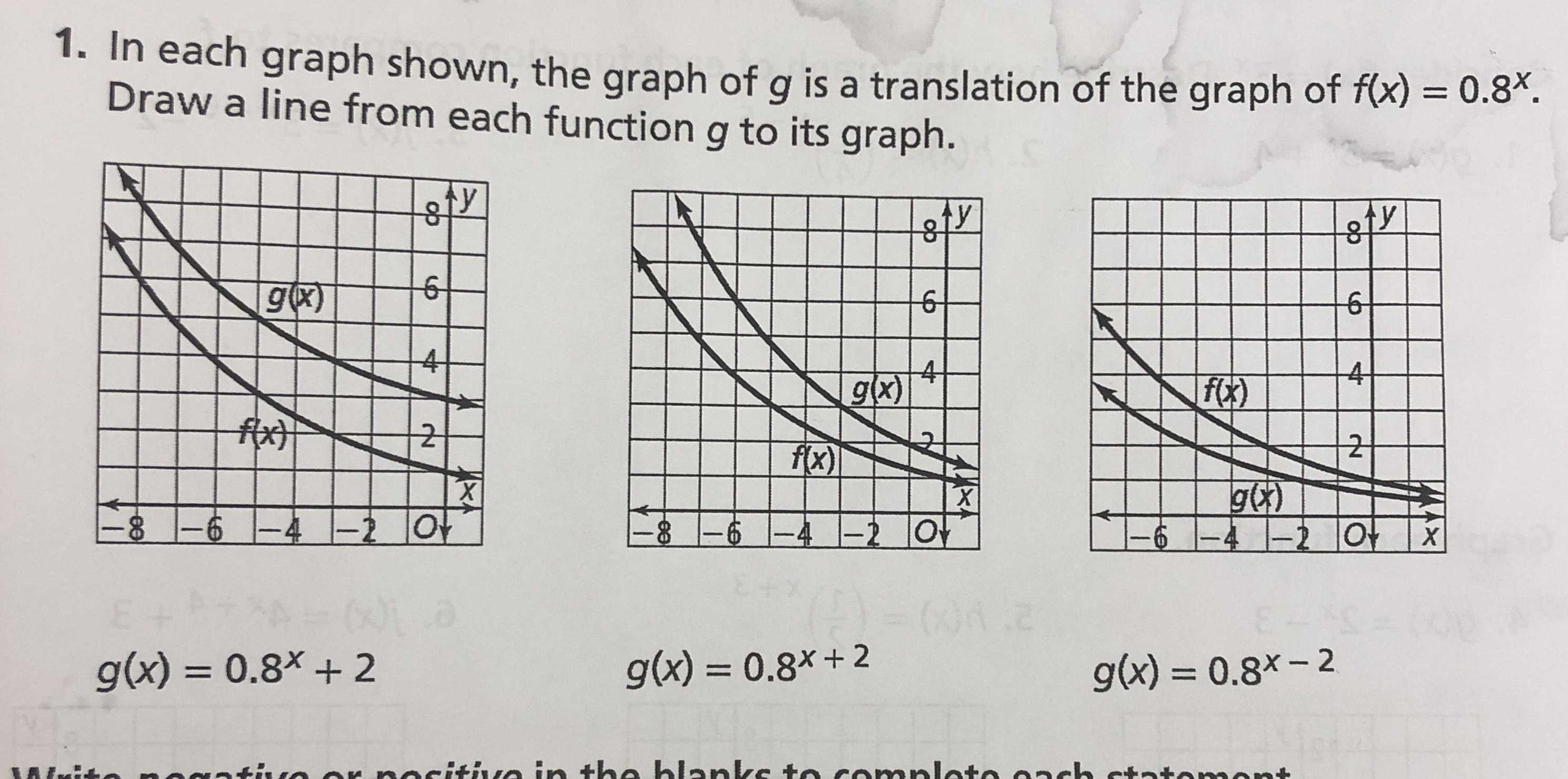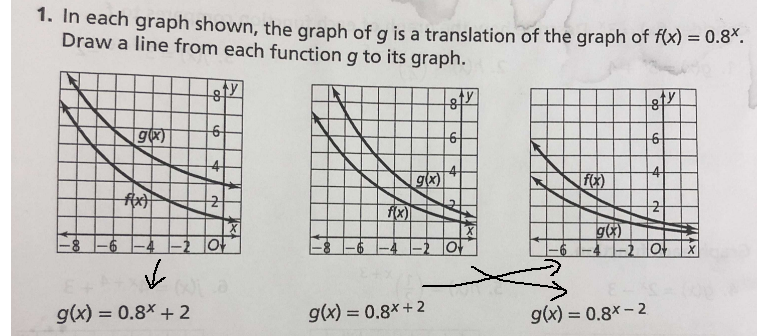### ¿Todavía tienes preguntas de matemáticas?

Pregunte a nuestros tutores expertos
Algebra
PreguntaIn each graph shown, the graph of $$g$$ is a translation of the graph of $$f ( x ) = 0.8 ^ { x }$$

Draw a line from each function $$g$$ to its qraph. $$g ( x ) = 0.8 ^ { x } + 2 \quad g ( x ) = 0.8 ^ { x + 2 } \quad g ( x ) = 0.8 ^ { x - 2 }$$